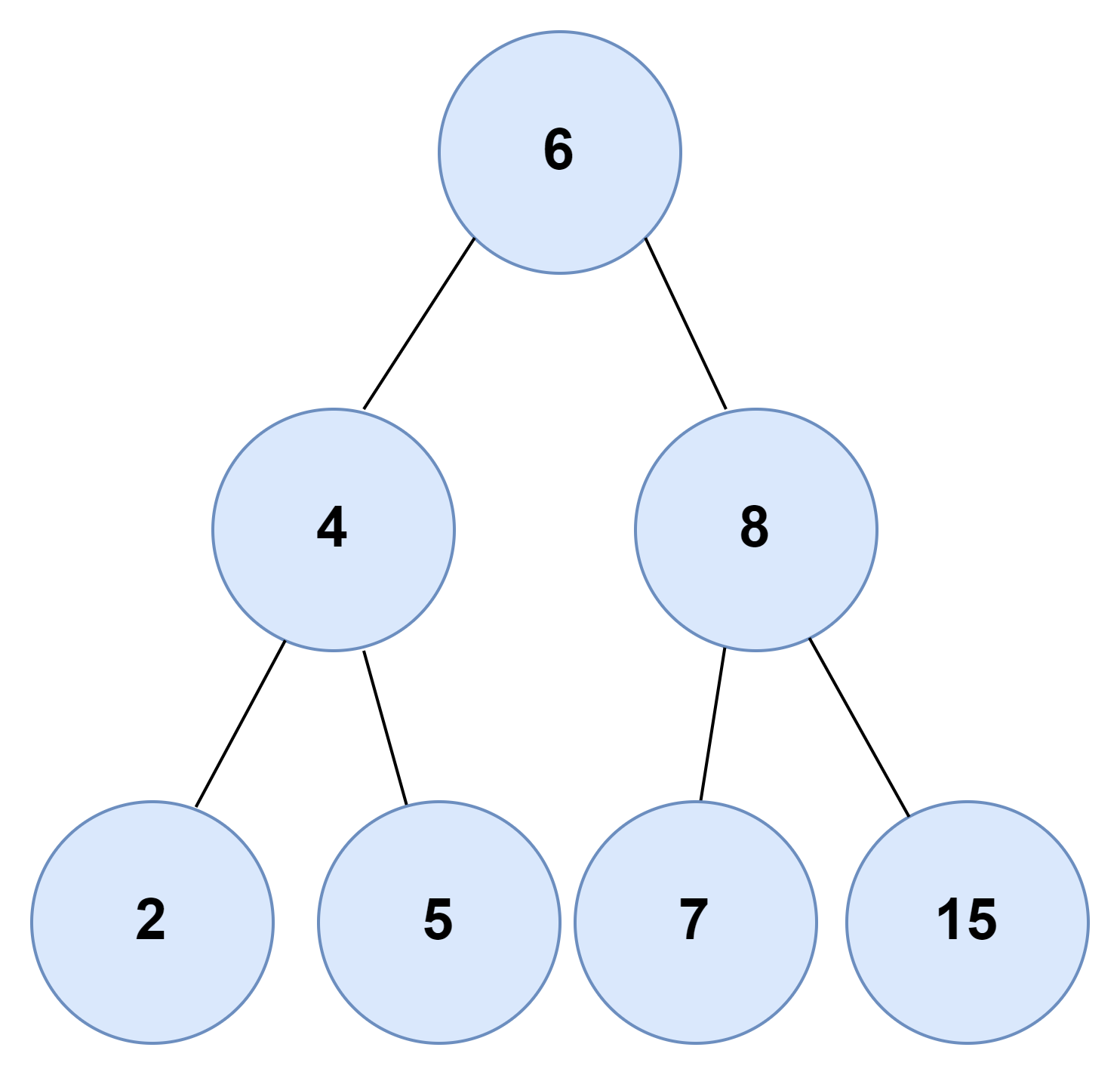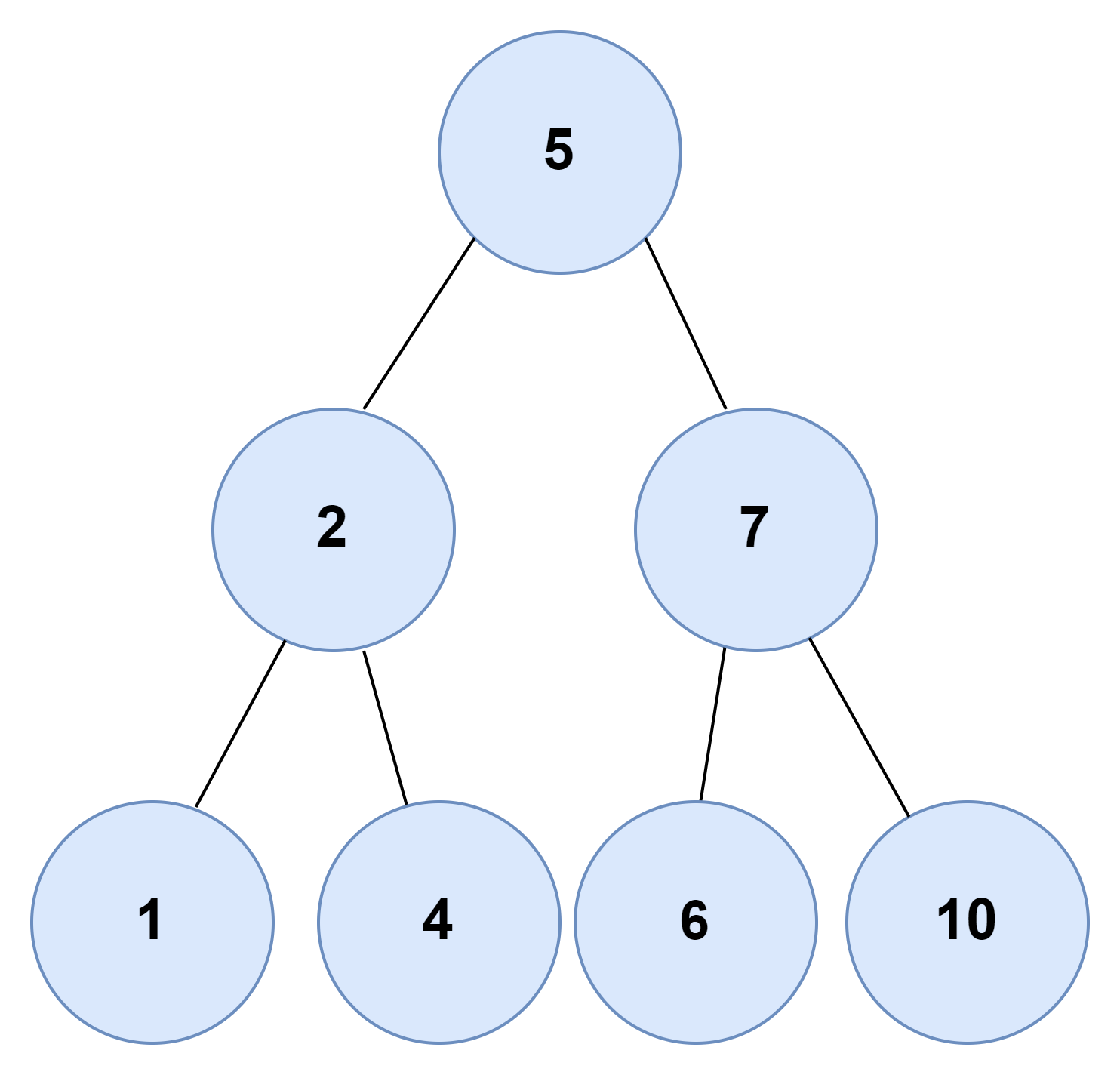# Number of pairs with a given sum in a Binary Search Tree

## Introduction

This blog will discuss the solution to the problem to find the number of pairs with a given sum in a binary search tree. Before we deep dive into the solution of this problem, we should look at some examples to have a better understanding of this problem.

### Sample Examples

Input: X = 15Output: Total number of pairs: 1

The possible pairs are: {7, 8}

Input: X = 7Output: Total number of pairs: 2

The possible pairs are: {{2, 5}, {1, 6}}

## Brute Force Approach

The brute force approach of this problem to find the number of pairs with a given sum in a binary search tree is to hash all the node values of the bst in the map. Then we will find pair by checking the map. For example, consider the value of given sum as x and suppose two nodes have values as a and b are present in the bst; since we have hashed all the node values of the bst beforehand, we will iterate in the map. When we find a, we will first check if it is less than x or not. If it is, then we will add m[X-a] into our answer, and we will now change the value of both m[a] and m[b] to zero. We will repeat this process till we reach the end of the map.

### Algorithm

1. We will make a function inorder() which will take one argument, i.e., the tree's root. We will maintain a map and hash all the elements of the bst into the map while traversing the bst.
2. Now in the main code, we will traverse the map and now suppose the current element of the map is a. Now we will check if a is less than x and if it is, then we will add m[X-a] to our answer, and after that, we will change the values of both m[a] and m[b] to zero.
3. After the map iteration is over, we will print our answer.

### Code in C++

``````// C++ implementation to find the number of pairs with a given sum in a binary search tree
#include <bits/stdc++.h>
using namespace std;

// Structure of each node of BST
struct Node
{
int key;
struct Node *left, *right;
};

// Function to create a new BST node
Node *TreeNode(int data)
{
Node *temp = new Node();
temp->key = data;
temp->left = temp->right = NULL;
return temp;
}

// Function to insert node in bst
struct Node *insert(struct Node *node, int key)
{
// If the tree is empty, then return a new node
if (node == NULL)
return TreeNode(key);

// Otherwise, recur down the tree
if (key < node->key)
node->left = insert(node->left, key);
else if (key > node->key)
node->right = insert(node->right, key);

// Return the (unchanged) node pointer
return node;
}

// to hash the elements of the bst
map<int, int> m;

// Function to count pairs with given sum
void inorder(struct Node *root)
{
// base case
if (!root)
return;

// hashing the current node in the map
m[root->key]++;

inorder(root->left);

inorder(root->right);
}
int main()
{

/* Formation of BST 1
5
/ \
2   7
/\   /\
1  4 6  10
*/

struct Node *root1 = NULL;
root1 = insert(root1, 5);
insert(root1, 2);
insert(root1, 1);
insert(root1, 4);
insert(root1, 7);
insert(root1, 6);
insert(root1, 10);

int x = 7, ans = 0;
inorder(root1);
for (auto y : m)
{
// if x is greater than the element
if (x > y.first)
{
// we will add the hash value of (x-y.first)
ans += m[x - y.first];
m[x - y.first] = 0;
m[y.first] = 0;
}
}
cout << "The total number of pairs are:" << ans << endl;

return 0;
}``````

Output:

``Output: Total number of pairs are: 2 ``

#### Complexity Analysis

Time Complexity: O(N)

Since we are doing in order traversal of the bst and inorder traversal can be done in O(N), the time complexity is O(N).

Space Complexity: O(N)

Since we are using a map to store the frequencies of all the nodes of the bst, therefore, the space complexity is O(N).

## Optimized Approach

The difference between the brute force approach and this approach is that we will be able to solve this problem using less space with this one space-efficient approach.

So the idea is to use the two-pointer approach on Bst. As the name suggests, we will maintain two pointers, one for forward iteration and another for backward iteration. Now, let's suppose the value of nodes at which they are pointing are x1 and x2.

We will first check if x1 + x2 == X then we will increase the count by 1 if x1 + x2 < X then we will move the forward iterator to the next node else if x1 + x2 > X then we will move the backward iterator to the previous node. We will repeat this process till both pointers point at the same Node.

### Algorithm

1. We will create two pointers, one named forward and the other named backward, and let's say they point at nodes x1 and x2, respectively.
2. Now at each iteration,
• If x1 + x2 == X then we will increase the count
• If x1 + x2 <= X, then we will make a forward pointer point to the next node
• If x1 + x2 > X, then we will make a backward pointer point to the previous Node
3. We will keep repeating these steps until the pointer points to the same Node.

### Code in C++

``````// C++ implementation to find the number of pairs with a given sum in a binary search tree
#include <bits/stdc++.h>
using namespace std;

// Structure of each node of BST
struct Node
{
int key;
struct Node *left, *right;
};

// Function to create a new BST node
Node *TreeNode(int data)
{
Node *temp = new Node();
temp->key = data;
temp->left = temp->right = NULL;
return temp;
}

// Function to insert node in bst
struct Node *insert(struct Node *node, int key)
{
// If the tree is empty, then return a new node
if (node == NULL)
return TreeNode(key);

// Otherwise, recur down the tree
if (key < node->key)
node->left = insert(node->left, key);
else if (key > node->key)
node->right = insert(node->right, key);

// Return the (unchanged) node pointer
return node;
}

// Function to find a pair
int countPairs(Node* root, int x)
{
// Stack to store nodes for both forward and backward pointers
stack<Node *> forward, backward;

// Forward iterator
Node* x1 = root;
while (x1 != NULL)
forward.push(x1), x1 = x1->left;

// Backward iterator
Node *x2 = root;
while (x2 != NULL)
backward.push(x2), x2 = x2->right;

int ans = 0;
Node *curr = NULL;
// two pointer technique
while (forward.top() != backward.top()) {
// To store the value of nodes
int x1 = forward.top()->key;
int x2 = backward.top()->key;

// if pair is valid then increase the count
if (x1 + x2 == x)
ans++;

// move the forward iterator
if (x1 + x2 <= x) {
curr = forward.top()->right;
forward.pop();
while (curr != NULL)
forward.push(curr), curr = curr->left;
}

// move the backward iterator
else {
curr = backward.top()->left;
backward.pop();
while (curr != NULL)
backward.push(curr), curr = curr->right;
}
}

return ans;
}
int main()
{

/* Formation of BST 1
6
/ \
3   8
/\   /\
2  4 7  9
*/

struct Node *root = NULL;
root = insert(root, 6);
insert(root, 3);
insert(root, 2);
insert(root, 4);
insert(root, 8);
insert(root, 7);
insert(root, 9);

int x= 11;
cout << "The total number of pairs are: " << answer << endl;

return 0;
}``````

Output:

``Output: Total number of pairs are: 3 ``

#### Complexity Analysis

Time Complexity: O(N)

Since we are doing in order traversal of the bst and inorder traversal can be done in O(N), the time complexity is O(N).

Space Complexity: O(H)

The space complexity is O(H), where H is the height of the bst.

Q1. What is the searching time complexity in a BST?

Ans. On average, the worst-case time complexity is O(Logn), and in the worst case, it’s O(n), i.e., the case of a skewed BST where ‘n’ is the number of nodes in the BST.

Q2. What are leave nodes in a tree?

Ans. Leave nodes of a tree are those nodes that don’t have any children, and both the pointers of the nodes point to null.

Q3. What is a binary search tree?

Ans. A binary search tree is a sorted binary tree in which all the nodes of the binary tree have a value greater than their left child node and a value smaller than their right child node.

## Key takeaways

In this article, we discussed the problem to find the number of pairs with a given sum in a binary search tree. We have discussed two approaches for this problem: the brute force approach and the efficient approach; the difference between the brute force approach and the efficient approach is that the efficient approach has better space complexity. We hope you have gained a better understanding of the solution to this problem and, now it is your responsibility to solve the problem and try some new and different approaches to this problem.

You can learn more about binary search trees hereUntil then, Keep Learning and Keep Coding and practicing on Code studio

.